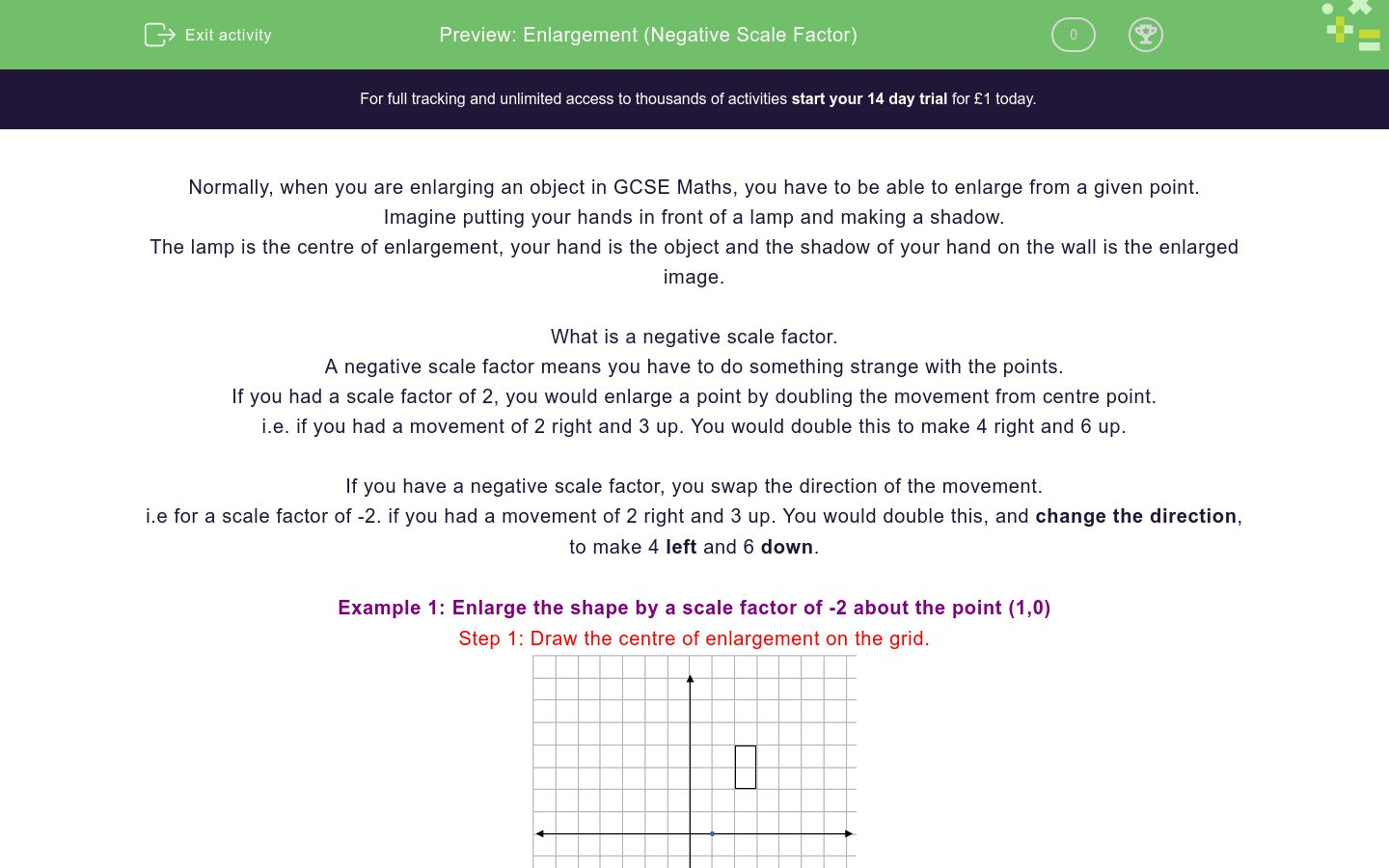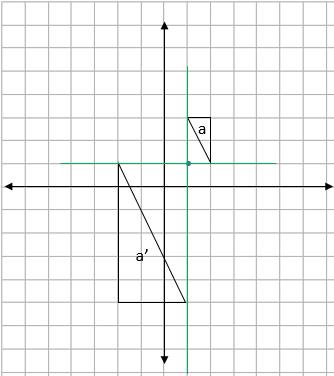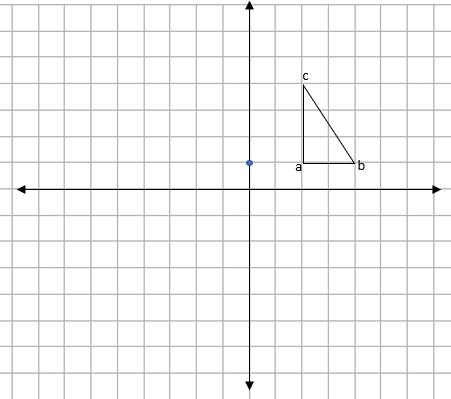# Enlargement (Negative Scale Factor)

In this worksheet, students practise enlarging a shape from a given point with a negative scale factor.Key stage:  KS 4

GCSE Subjects:   Maths

GCSE Boards:   AQA, Eduqas, Pearson Edexcel, OCR

Curriculum topic:   Geometry and Measures, Congruence and Similarity

Curriculum subtopic:   Properties and Constructions, Plane Isometric Transformations

Difficulty level:### QUESTION 1 of 10

Normally, when you are enlarging an object in GCSE Maths, you have to be able to enlarge from a given point.

Imagine putting your hands in front of a lamp and making a shadow.

The lamp is the centre of enlargement, your hand is the object and the shadow of your hand on the wall is the enlarged image.

What is a negative scale factor.

A negative scale factor means you have to do something strange with the points.

If you had a scale factor of 2, you would enlarge a point by doubling the movement from centre point.

i.e. if you had a movement of 2 right and 3 up. You would double this to make 4 right and 6 up.

If you have a negative scale factor, you swap the direction of the movement.

i.e for a scale factor of -2. if you had a movement of 2 right and 3 up. You would double this, and change the direction, to make 4 left and 6 down.

Example 1: Enlarge the shape by a scale factor of -2 about the point (1,0)

Step 1: Draw the centre of enlargement on the grid.Doing this means you wont forget where you're enlarging from.

Step 2: Find the movement from the centre to one of the points on the shape.We can see that to get to the bottom left corner, we move 1 right and 2 up

Step 3: Multiply this movement by the Scale Factor.

Our scale factor is -2, so this movement doubles and changes direction to 2 left and 4 down.

Step 4: Plot this point on the diagram.

When you are plotting this point, it is vital that you count from the centre of enlargement.Step 5: Repeat steps 2 - 4 for the other corners of the shape.Step 6: Draw the enlarged shape and label the image and enlargement.Example 2: Shape A is enlarged to shape B. Find the scale factor and centre of enlargement.Finding the Scale Factor.

All we have to do here is to look at one of the pairs of sides. If we use the top of each shape, shape A is 1cm long and shape B is 3 cm long.

Because the centre is between the shapes and the shape has turned around , we must have a negative scale factor

This gives a scale factor of -3

.

Finding the centre of enlargement.

To find this, we join up two pairs of matching corners with striaght lines and see where they join up.

You need to be really careful that you match up the correct pairs of coners, remember that the shape has flipped upside down.We can see here that the two lines join up at the point (1,1). This is the centre of enlargement.

A negative SF will mean that the enlarged shape will be on the ...

Rectangle ABCD is enlarged by SF -2 around the point (0,0).Find the coordinates of a'

Rectangle ABCD is enlarged by SF -2 around the point (0,0).Find the coordinates of d'

Rectangle ABCD is enlarged by SF -3 around the point (2,0).Find the coordinates of b'

Rectangle ABCD is enlarged by SF -3 around the point (2,0).Find the coordinates of c'

Shape a is enlarged around the point (0,0). What is the SF of the enlargement?Shape a is enlarged to shape a'. Find the SF and centre of the enlargement.Enlarging by a negative scale factor will always change the size of the object.

True

False

The triangle abc is enlarged with a SF of  -2 about the point (0,1).What are the coordinates of a',b' and c'

 Coordinate a' b' c'

The rectangle abcd is enlarged with a SF of  -1/2  about the point (-1,0).What are the coordinates of a',b',c' and d'

 Coordinate a' b' c' d'
• Question 1

A negative SF will mean that the enlarged shape will be on the ...

EDDIE SAYS
A negative scale factor does something very similar to a rotation of 180°. The enlargement will always be on the opposite side of the centre of enlargement to the original shape.
• Question 2

Rectangle ABCD is enlarged by SF -2 around the point (0,0).Find the coordinates of a'

(-2,-2)
EDDIE SAYS
To get from the centre (0,0) to point A is a movement of 1 right and 1 up. If we double this and change directions (SF -2), we get a movement of 2 left and 2 down. We then do this movement from the centre to get the point a'.
• Question 3

Rectangle ABCD is enlarged by SF -2 around the point (0,0).Find the coordinates of d'

(-2,-4)
EDDIE SAYS
To get from the centre (0,0) to point D is a movement of 1 right and 2 up. If we double this and change directions (SF -2), we get a movement of 2 left and 4 down. We then do this movement from the centre to get the point d'.
• Question 4

Rectangle ABCD is enlarged by SF -3 around the point (2,0).Find the coordinates of b'

(-1,-3)
EDDIE SAYS
To get from the centre (2,0) to point B is a movement of 1 right and 1 up. If we triple this and change directions (SF -3), we get a movement of 3 left and 3 down. We then do this movement from the centre to get the point b'.
• Question 5

Rectangle ABCD is enlarged by SF -3 around the point (2,0).Find the coordinates of c'

(10,9)
EDDIE SAYS
To get from the centre (2,0) to point C is a movement of 1 right and 2 up. If we triple this and change directions (SF -3), we get a movement of 3 left and 6 down. We then do this movement from the centre to get the point c'.
• Question 6

Shape a is enlarged around the point (0,0). What is the SF of the enlargement?-2
EDDIE SAYS
If we count the lengths of the two shapes, we see that the lengths are doubled. With the enlargement being the other way around, we can say that this is a negative scale factor. This gives a scale factor of ...
• Question 7

Shape a is enlarged to shape a'. Find the SF and centre of the enlargement.EDDIE SAYS
Did I manage to catch you out? Did you notice that the shape was getting smaller? This is actually a scale factor of - 1/2
• Question 8

Enlarging by a negative scale factor will always change the size of the object.

False
EDDIE SAYS
It's down to a single word. Will it always change size? What about a scale factor of -1?
• Question 9

The triangle abc is enlarged with a SF of  -2 about the point (0,1).What are the coordinates of a',b' and c'

 Coordinate a' b' c'
EDDIE SAYS
The biggest mistake people make here is to not notice it is a negative SF. Remember this changes the direction you are counting in. For example, to get the coordinate of c'. We find the movement from our centre (0,1) to the point C - This is 2 right and 3 up Doubling this and changing the direction (SF -2) gives a movement of 4 left and 6 down This gives the coordinate of c' as (-4,-5)
• Question 10

The rectangle abcd is enlarged with a SF of  -1/2  about the point (-1,0).What are the coordinates of a',b',c' and d'

 Coordinate a' b' c' d'
EDDIE SAYS
Did you notice the fractional negative this time? Don't forget that this means you are making the shape smaller. For example, to get the coordinate of c'. We find the movement from our centre (-1,0) to the point C - This is 2 left and 6 down Halving this and changing the direction (SF -1/2) gives a movement of 1 right and 3 up This gives the coordinate of c' as (0,3)
---- OR ----

Sign up for a £1 trial so you can track and measure your child's progress on this activity.

### What is EdPlace?

We're your National Curriculum aligned online education content provider helping each child succeed in English, maths and science from year 1 to GCSE. With an EdPlace account you’ll be able to track and measure progress, helping each child achieve their best. We build confidence and attainment by personalising each child’s learning at a level that suits them.

Get started